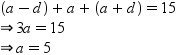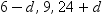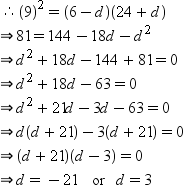# Test: Arithmetic And Geometric Progressions - 4

## 40 Questions MCQ Test Quantitative Aptitude for CA CPT | Test: Arithmetic And Geometric Progressions - 4

Description
Attempt Test: Arithmetic And Geometric Progressions - 4 | 40 questions in 40 minutes | Mock test for CA Foundation preparation | Free important questions MCQ to study Quantitative Aptitude for CA CPT for CA Foundation Exam | Download free PDF with solutions
QUESTION: 1

Solution:
QUESTION: 2

Solution:
QUESTION: 3

### If a, b-a, c-a are in G.P. and a=b/3=c/5 then a, b, c are in

Solution:
QUESTION: 4

The sum of term of the series 5+55+555+…..is

Solution:
QUESTION: 5

If a, b, (c+1) are in G.P. and a=(b-c)2 then a, b, c are in

Solution:
QUESTION: 6

If S1, S2, S3, ………Sn are the sums of infinite G.P.s whose first terms are 1, 2, 3……n and whose common ratios are 1/2, 1/3, ……1/(n+1) then the value of S1+S2+S3+ ……Sn is

Solution:
QUESTION: 7

If a, b, c are in G.P. then the value of (a-b+c)(a+b+c)2-(a+b+c)(a2+b2+c2) is given by

Solution:
QUESTION: 8

If a1/x=b1/y=c1/z and a, b, c are in G.P. then x, y, z are in

Solution:
QUESTION: 9

If a, b, (c+1) are in G.P. and a=(b-c)2 then a, b, c are in

Solution:
QUESTION: 10

If a, b, c are in G.P. then a2+b2, ab+bc, b2+c2 are in

Solution:
QUESTION: 11

If a, b, c are in G.P then the value of a (b2+c2)-c(a2+b2) is given by

Solution:
QUESTION: 12

If a, b, c are in G.P. then value of a2b2c2(a-3+b-3+c-3)-(a3+b3+c3) is given by

Solution:
QUESTION: 13

If a, b, c, d are in G.P. then a+b, b+c, c+d are in

Solution:
QUESTION: 14

If a, b, c are in A.P. a, x, b are in G.P. and b, y, c are in G.P. then x2, b2, y2 are in

Solution:
QUESTION: 15

If a, b, c, d are in G.P. then the value of (b-c)2+(c-a)2+(d-b)2-(a-d)2 is given by

Solution:
QUESTION: 16

If (a-b), (b-c), (c-a) are in G.P. then the value of (a+b+c)2-3(ab+bc+ca) is given by

Solution:
QUESTION: 17

If a, b, c, d are in G.P. then (a-b)2, (b-c)2,(c-a)2 are in

Solution:
QUESTION: 18

If a, b, x, y, z are positive numbers such that a, x, b are in A.P. and a, y, b are in G.P. and z=(2ab)/(a+b)then

Solution:
QUESTION: 19

If a, b-a, c-a are in G.P. and a=b/3=c/5 then a, b, c are in

Solution:
QUESTION: 20

If a, b, c, d are in G.P. then the value of b(ab-cd)-(c+a)(b2-c2) is ________

Solution:
QUESTION: 21

The least value of n for which the sum of n terms of the series 1+3+32+………..is greater than 7000 is _________.

Solution:
QUESTION: 22

If a, b, c are in A.P. and x, y, z in G.P. then the value of (xb.yc.za) ÷ (xc.ya.zb) is _________.

Solution:
QUESTION: 23

If a, b, c are the pth, qth and rth terms of a G.P. respectively the value of aq-r. br-p. cp-q is ___________.

Solution:
QUESTION: 24

If a, b, c are in G.P. then the value of a(b2+c2)-c(a2+b2) is __________

Solution:
QUESTION: 25

If S be the sum, P the product and R the sum of the reciprocals of n terms in a G.P. then P is the ________ of Sn and R-n.

Solution:
QUESTION: 26

If the sum of three numbers in G.P. is 21 and the sum of their squares is 189 the numbers are __________.

Solution:
QUESTION: 27

The sum of n terms of the series 7+77+777+……is

Solution:
QUESTION: 28

If a, b, c, d are in G.P. then the value of (ab+bc+cd)2-(a2+b2+c2)(b2+c2+d2)is ______.

Solution:
QUESTION: 29

If 1+a+a2+………∞=x and 1+b+b2+……∞=y then 1+ab+a2b2+………∞ = x is given by ________.

Solution:

Solution :- Given, x=1+a+a^2+......∞

Since this is a infinite G.P. series, where, (first term)=1 and (common difference)=a,

So, x = 1/(1−a)

⇒ x−ax=1

⇒ ax=x−1

⇒ a=(x−1)/x

Similarly, y=1+b+b^2 +......∞ is a infinite G.P. series, where, (first term)=1 and

(common difference)=b,

So, y = 1/(1−b)

⇒ y−by=1

⇒ by=y−1

⇒ b=(y−1)/y

And now,

L.H.S.=1 + ab + a^2b^2 + ....∞

= 1/(1−ab) (infinte G.P. series where (first term)=1 and (common difference)=ab

= 1/{1−(x−1/x)(y−1/y)}

= xy/(xy−xy+x+y−1)

= (xy)/(x+y−1)

QUESTION: 30

Sum upto ∞ of the series 1/2+1/32+1/23+1/34+1/25+1/36+……is

Solution:
QUESTION: 31

If a, b, c are in A.P. and x, y, z in G.P. then the value of xb-c. yc-a. za-b is ______.

Solution:
QUESTION: 32

If the sum of three numbers in G.P. is 35 and their product is 1000 the numbers are _________.

Solution:
QUESTION: 33

Three numbers whose sum is 15 are A.P. but if they are added by 1, 4, 19 respectively they are in G.P. The numbers are _________.

Solution:

Let the given numbers in A.P. be a – d,  ad.

According to question,Hence, the numbers are 5 – d, 5, 5 + d.

Adding 1, 4 and 19 in first, second and third number respectively, we getSince these numbers are in G.P.Hence the numbers are

26, 5, –16  or  2, 5, 8.

QUESTION: 34

n(n-1)(2n-1) is divisible by

Solution:

For n=1

n(n+1)(2n+1) = 6, divisible by 6.

Let the result be true for n=k

Then, k(k+1)(2k+1) is divisible by 6.

So k(k+1)(2k+1) =6m   (1)

Now to prove that the result is true for n=k+1

That is to prove, (K+1)(k+2)(2k+3) is divisible by 6.

(K+1)(k+2)(2k+3)=(k+1)k(2k+3)+(k+1)2(2k+3)=(k+1)k(2k+1)+(k+1)k2+(k+1)2(2k+3)

=6m+2(k+1)(k+2k+3)      using (1)

=6m+2(k+1)(3k+3)

=6m +6(k+1)(k+1)=6[m+(k+1)^2]

So divisible by6.

QUESTION: 35

The value of n2++2n[1+2+3+…+(n-1)] is

Solution:
QUESTION: 36

The sum of n terms of the series 13/1+(13+23)/2+(12+22+33)/3+……is

Solution:
QUESTION: 37

3n-2n-1 is divisible by

Solution:
QUESTION: 38

The sum of n terms of the series 3+6+11+20+37+……… is

Solution:
QUESTION: 39

The nth terms of the series is 1/(4.7)+1/(7.10)+1/(10.13)+………is

Solution:
QUESTION: 40

If the third term of a G.P. is the square of the first and the fifth term is 64 the series would be __________.

Solution:Use Code STAYHOME200 and get INR 200 additional OFF Use Coupon Code